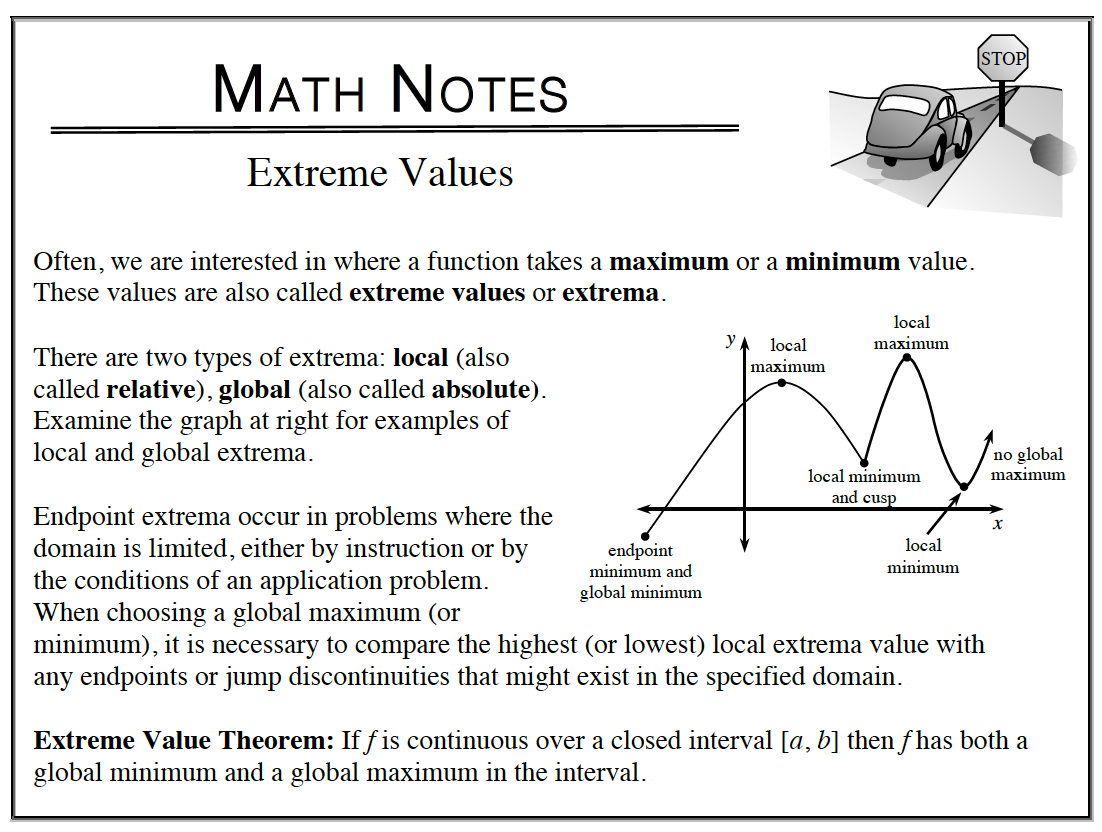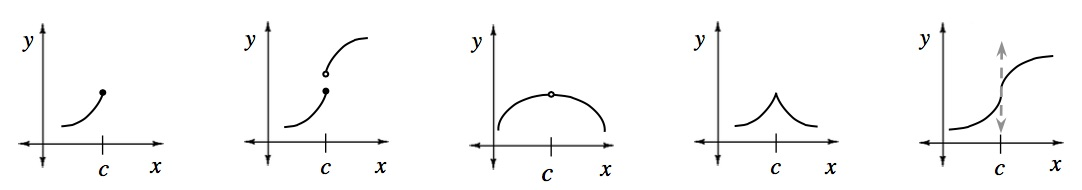### Home > APCALC > Chapter 6 > Lesson 6.2.1 > Problem6-63

6-63.

Write the first and second derivatives for the function below and use them to test for extrema over the given interval. Remember to check the endpoints! Homework Help ✎

$y = 2 \sin(x) + 3 \cos(x) \text{ over } [0, π]$

This problem is asking you to find EXTREME VALUES on a closed domain.
Extreme values (global maxima and global minima) can exist where $y' = 0$ or where $y' =$ DNE.Locate extrema candidates where y' = 0. Remember to only consider candidates within the given domain: [0, π].
$y' = 2\cos(x) − 2\sin(x) = 2(\cos(x) − \sin(x))$
$0 = 2(\cos(x) − \sin(x))$
$\cos(x) = \sin(x)$

$x=\frac{\pi}{4}$

Locate more extrema candidates where y' = DNE. In other words, where is y non-differentiable? Are there endpoints, jumps, holes, cusps, or vertical tangents? If so, these are extrema candidates. There are endpoint candidates at $x = 0$ and $x = π$.Three extrema candidates have been identified.

$x=0,\ x=\frac{\pi}{4}\text{, and }x=\pi.$

Test each candidate to see who has the most extreme (highest and lowest) y-values.
The 'winners' are the global max and the global min.

$y(0) =$ _________

$y\left (\frac{\pi}{4} \right )=\underline{\ \ \ \ \ \ \ \ \ \ \ \ \ }$

$y(π) =$ _________